# Charting Specifications and Limits for Excel 2003, 2007 and 2010

Have you ever wondered how many data series you can actually add to an Excel chart?  Well there are limits.  Recently an Excel Client was having problems creating a chart with more than 34 categories?  I suggested that her chart problems were not a limit of Excel, but perhaps user error.  So I looked into what the actual Excel Limits are for Charts and Graphs.

Here are the Excel chart limits for Excel 2003, Excel 2007 and Excel 2010 compared side by side: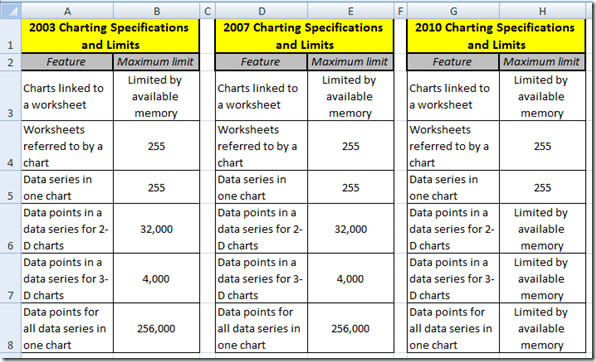Notice that there is are limits to the number of data points or ultimately data categories in Excel 2010 as long as your computer has enough memory.  However, in Excel 2003 and Excel 2007, you are limited to 32,000 data points for 2-D charts.

So I tested it out.  I created a set of data with dates from January 1, 1900 and added one to the next date and then created a data column that increases by one just so we can see it on the chart more easily.  Here is what the data looks like and I copied it down to row 32009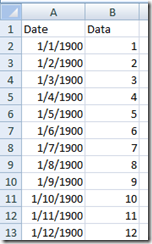I highlighted the entire range and got this error in Excel 2003: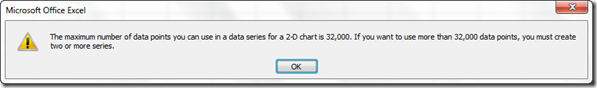So I changed the range to just under 32,000 data points and the chart appeared as such: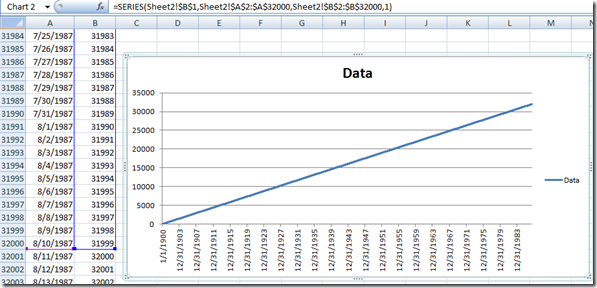Okay, so the Excel Error stated that if you want more than 32,000 data points, use 2 or more series, so I did that and it works.  So I kept adding series of 32,000 data points and it even works for 10 series of 32,000 data points: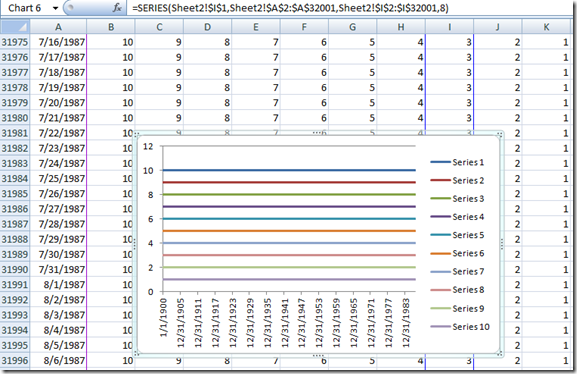That is 320,000 data points in one chart.  Wait a minute??  I thought the Excel Limits for 2007 were 256,000 data points?

Strange, but maybe the limits are not as hard and fast as described between 2007 and 2010.  Perhaps this help document wasn’t proofed or my version of Excel 2007 was changed in a recent update?  Do you know?

Okay, so then I thought if it is more like Excel 2010, then perhaps I am only limited to by my computer’s memory.  So I created a chart with 32,000 data points for 255 columns (data series).   It took about 5 minutes for Excel to create this chart, but it worked!!!

As you can see in my copy of Excel 2007, the data series is number 255 and the range for this data series is IV2:IV32001.  That is 8.16 MILLION data points on one chart.  Excel is AMAZING!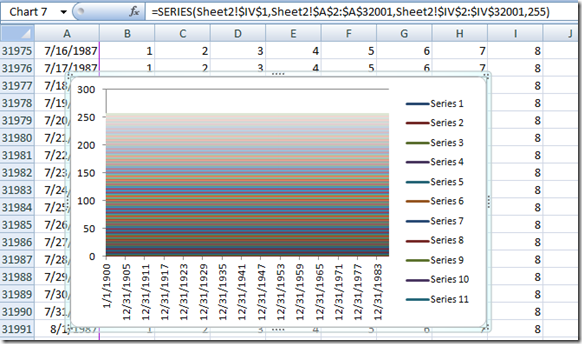Since this chart only had 255 data series, I tried to do more than 255 data series in an Excel Chart and it did give me that error: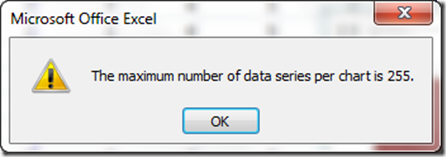So you can have millions of data points, but only 255 data series even if your computer has the memory to handle it.

Here are links to Excel Charting Specifications and Limits:

More than 255 Series?

So let me know, would you ever need more than 255 data series and Excel Chart?

Would you ever need more than 256,000 data points in a Graph?

Can the human eye detect any of these differences?